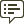Community Profile### MathWorks

Last seen: Today 2014 以来アクティブ

Professional Interests:

#### Statistics

All
••••••••••••バッジを表示

#### Content Feed

Creating a polar bar chart or circumplex chart (not a histogram)
See polarhistogram. If you have data suitable for a bar plot and, therefore, you don't need to compute the bins and bar heights...

1日 前 | 1

Take 102 Elements Equally Spaced of an Existing Array
> Is there a way to solve this issue? No. 991 is not divisible by 102. The following is a 102 vector starting with 1 and end...

1日 前 | 1

| 採用済み

Readtable don't work correctly if 'Range' > 'A250'
That doesn't sound good. If you manually grab the import options, that addresses the problem. opts = spreadsheetImportOptio...

1日 前 | 0

| 採用済み

how can I plot a graph over an image in Matlab?
Plot the image using image or imagesc or some other image function that allows you to specify the x and y values of the image. ...

3日 前 | 2

Accessing cell array contents
See cellfun In this example, the cell array is named myCellArray and the variable is named myVar. y = cellfun(@(A)A.myVar(en...

3日 前 | 2

Converting wind/wave data to hourly data by interpolation
If the durations are consecutive, then I suggest creating an time stamps based on the durations which allows you to use retime t...

3日 前 | 0

| 採用済み

How to plot correlation coefficient matrix plot?
Assuming you already have the correlation matrix, use heatmap. The upper triangle can be filled with NaNs. S = load('Data_Can...

3日 前 | 1

A green arrow in an m-file
You are in debug mode. The green arrows hows the line at which MATLAB is currently paused. how did I get here? There a...

4日 前 | 0

| 採用済み

+60k Lines on one plot - Too slow
Another idea is to plot your 60k lines as a 2D density plot (initially suggested in this thread). Pros: 1 graphics object, l...

7日 前 | 0

| 採用済み

How to convert fprintf from single row to multiple rows?
I assume you want to show each numeric value in a new line. To do that, add a new line control character where you want to inse...

8日 前 | 0

how I show all the numbers in y axes
> its important to me that all the numbers appears even these small changes Is your concern that readers will not believe that ...

8日 前 | 0

Sorting table row variables with number and letters
Use TableC = sortrows(TableB,'RowNames') This option also appears in the OP's 2017b documentation.

8日 前 | 1

| 採用済み

What does a(a<0) = 0 mean in matlab?
> What does a(a<0) = 0 mean? a can be a scalar or an array. This replaces all values in a that are less than 0 with 0. Ex...

10日 前 | 1

Box plot into figure with another graphs
MATLAB's boxplot and boxchart are designed to create box plots from the raw data. boxplot(Predictions_sorted) % or boxchar...

10日 前 | 0

| 採用済み

Standard deviation in 3D array from 1st to nth number array
To cumulatively compute the std across dimension 3, data = rand(6, 493, 6000); RN = zeros(size(data,[1,3])); for i = 1 : si...

10日 前 | 0

"Graphics timeout occurred"
Can you reproduce this issue? If so, sharing the steps that reproduce this would be helpful. Nevertheless, please report thi...

12日 前 | 0

| 採用済み

how to compare column values of a matrix with a column vector of different size?
Load data detected = [56 40 33 31 28 13 10 1]; M_transmision = [1 40 -84 -638 6...

12日 前 | 0

| 採用済み

Convert double to categorical array
The function categorical can convert continuous data into categorical data. However, this function cannot categorize two numbers...

16日 前 | 0

| 採用済み

'Value' must be double scalar within the range of 'Limits'

16日 前 | 1

| 採用済み

Best way to analyze two waveform signals
Hello @Austin Bollinger, looks like @Jonas has made some good recommendations. I'll add another perspective. My grad school r...

16日 前 | 0

| 採用済み

Creating callable function with prompts
txt = input(prompt,"s") Include the "S" in argument 2 to return the entered text without evaluating it. > created a bunch o...

17日 前 | 0

Set xaxis of imagesc with scaled values
Specify x and y values using imagesc(x,y,C) I think this is what you're looking for C = rand(20,400); % Replace this with your...

| 採用済み

change focus in app desinger
> Is there a way to put the cursor in the editbox without click the box Yes, as of MATLAB R2022a. See the focus function whic...

2ヶ月 前 | 0

Why is my p-value matrix after using corrcoef filled with NaN?
First check whether you have NaNs in your inputs. If so, that explains why there are NaNs in your outputs. If you do not have...

2ヶ月 前 | 0

| 採用済み

Missing legend entries in plotyy
Use yyaxis instead of plotyy. Instead of using plotyy(x1,y1,x2,y2) use yyaxis left plot(x1,y1) yyaxis right plot(x2,y2...

2ヶ月 前 | 0

How to pick maximum value in group?
data = [0.74 0.66 0.58 0.47 0.606 0.705 0.76]; group = [6 6 5 6 5 6 6]; groupsummary(data(:),group(:),'max')...

2ヶ月 前 | 0

| 採用済み

Using for loop to find values greater than a number in each column.
It's a pitty that the homework assignment requires using a loop since this can be solved in one line of code. y = mat > mean(m...

2ヶ月 前 | 0

Highlighted

National Volunteer Week: Contribute to the MATLAB Central Community
<<https://www.mathworks.com/matlabcentral/discussions/uploaded_files/11465/data>>  *20 minutes makes a difference* I...

3ヶ月 前 | 13how to create one plot with several contours (corner plot)
As KSSV recommended, tiledlayout is the way to go. tcl = tiledlayout(3,3,'TileSpacing','none'); tileIdx = [1,4,5,7,8,9]; %...

3ヶ月 前 | 0